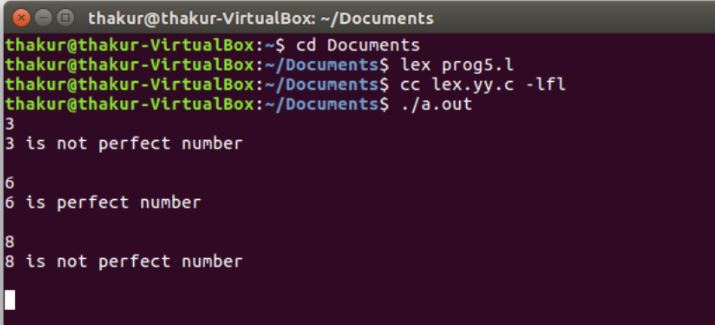Related Articles

# Lex program to check perfect numbers

• Difficulty Level : Expert
• Last Updated : 30 Apr, 2019

Lex is a computer program that generates lexical analyzers and was written by Mike Lesk and Eric Schmidt. Lex reads an input stream specifying the lexical analyzer and outputs source code implementing the lexer in the C programming language.

Description: Perfect number, a positive integer that is equal to the sum of its proper divisors, for example: 6 = 1+2+3.

Examples:

```Input: 3
Output: 3 is not perfect number

Input: 6
Output: 6 is perfect number ```

Implementation:

 `/*Lex program to check perfect numbers*/`` ` `%``{``#include ``    ``void` `check(``char``*);``    ``%``}`` ` `/*Rule Section*/``% %``        ``[0 - 9]``    ``+ check(yytext);``% % ``int` `main()``{``    ``// the input stream pointer``    ``extern` `FILE``* yyin;`` ` `    ``// open a file handle to a particular file``    ``yyin = ``fopen``(``"num"``, ``"r"``);`` ` `    ``// The function that starts the analysis``    ``yylex();``    ``return` `0;``}``void` `check(``char``* a)``{``    ``int` `len = ``strlen``(a), i, num = 0;`` ` `    ``for` `(i = 0; i < len; i++)``        ``num = num * 10 + (a[i] - ``'0'``);`` ` `    ``int` `x = 0, temp = num;``    ``for` `(i = 1; i < num; i++) {``        ``if` `(num % i == 0)``            ``x = x + i;``    ``}``    ``if` `(x == temp)``        ``printf``(``"%d is perfect number \n"``, num);`` ` `    ``else``        ``printf``(``"%d is not perfect number \n"``, num);``}`

Output:We provide nothing but the best curated videos and practice problems for our students. Check out the C Foundation Course and master the C language from basic to advanced level. Wait no more, start learning today!

My Personal Notes arrow_drop_up